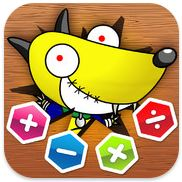Select PageNumerosity is a new maths app for iPad just released in the iTunes App Store from the Thoughtbox company. Its purpose is to reinforce learning in the four main mathematical operations operations. I asked one of my pupils to review the app for me and these are his thoughts:

My teacher asked my to do a review on this new maths game he found on the iPad. The app is called Numerosity. Numerosity is a game where this dog called Scruffy has lots of problems with maths so some of his friends started Numerosity to help. When you start off in the game it shows a picture of Scruffy and beside him there are five hexagons, each one of them has a number inside. Each number means the next level, the levels are 1-5. The first level is to do with adding. It shows you two numbers for example 8 + 9 = ? At the bottom of the screen you have four answers and one of them is correct. You have about a minute to answer as many sums as possible. For each one you get right, you get some points and at the end there are three X’s. Each X is made from two bones and the higher your score, the more bones you will get. Level two is subtract, level three is multiply, and level four is dividing. Then level five is the trickiest. It shows you for example 9 + 9 ÷ 2. Some are easy but the other few are very very hard because you have to switch the sum around to be able to do the sum. I know that sounds hard but it is when you only have about 5 seconds to do it. So that is my review on the app Numerosity.